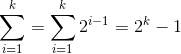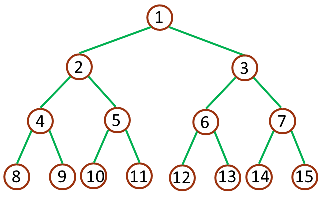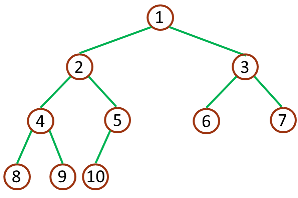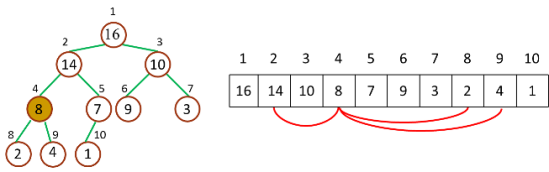# （4）堆排序 (Heap Sort)

2018-04-18 16:04 更新

## 算法原理

1. 不得不说说二叉树• 树的结点个数至少为 1，而二叉树的结点个数可以为 0
• 树中结点的最大度数没有限制，而二叉树结点的最大度数为 2
• 树的结点无左、右之分，而二叉树的结点有左、右之分2. 什么是堆？

• Parent(i) = floor(i/2)，i 的父节点下标
• Left(i) = 2i，i 的左子节点下标
• Right(i) = 2i + 1，i 的右子节点下标• 最大堆中的最大元素值出现在根结点（堆顶）
• 堆中每个父节点的元素值都大于等于其孩子结点（如果存在）

• 最小堆中的最小元素值出现在根结点（堆顶）
• 堆中每个父节点的元素值都小于等于其孩子结点（如果存在）

3. 堆排序原理

• 最大堆调整（Max-Heapify）：将堆的末端子节点作调整，使得子节点永远小于父节点
• 创建最大堆（Build-Max-Heap）：将堆所有数据重新排序，使其成为最大堆
• 堆排序（Heap-Sort）：移除位在第一个数据的根节点，并做最大堆调整的递归运算

• Parent(i) = floor((i-1)/2)，i 的父节点下标
• Left(i) = 2i + 1，i 的左子节点下标
• Right(i) = 2(i + 1)，i 的右子节点下标

``````/**
* 从 index 开始检查并保持最大堆性质
*
* @array
*
* @index 检查的起始下标
*
* @heapSize 堆大小
*
**/
function maxHeapify(array, index, heapSize) {
var iMax = index,
iLeft = 2 * index + 1,
iRight = 2 * (index + 1);

if (iLeft
iMax = iLeft;
}

if (iRight
iMax = iRight;
}

if (iMax != index) {
swap(array, iMax, index);
maxHeapify(array, iMax, heapSize); // 递归调整
}
}

function swap(array, i, j) {
var temp = array[i];
array[i] = array[j];
array[j] = temp;
}``````

``````/**
* 从 index 开始检查并保持最大堆性质
*
* @array
*
* @index 检查的起始下标
*
* @heapSize 堆大小
*
**/
function maxHeapify(array, index, heapSize) {
var iMax, iLeft, iRight;
while (true) {
iMax = index;
iLeft = 2 * index + 1;
iRight = 2 * (index + 1);
if (iLeft
iMax = iLeft;
}

if (iRight
iMax = iRight;
}

if (iMax != index) {
swap(array, iMax, index);
index = iMax;
} else {
break;
}
}
}

function swap(array, i, j) {
var temp = array[i];
array[i] = array[j];
array[j] = temp;
}``````

``````function buildMaxHeap(array, heapSize) {
var i,
iParent = Math.floor((heapSize - 1) / 2);

for (i = iParent; i >= 0; i--) {
maxHeapify(array, i, heapSize);
}
}``````

``````function heapSort(array, heapSize) {

buildMaxHeap(array, heapSize);

for (int i = heapSize - 1; i > 0; i--) {
swap(array, 0, i);
maxHeapify(array, 0, i);
}
}``````

## JavaScript 语言实现

``````function heapSort(array) {

function swap(array, i, j) {
var temp = array[i];
array[i] = array[j];
array[j] = temp;
}

function maxHeapify(array, index, heapSize) {
var iMax,
iLeft,
iRight;
while (true) {
iMax = index;
iLeft = 2 * index + 1;
iRight = 2 * (index + 1);

if (iLeft < heapSize && array[index] < array[iLeft]) {
iMax = iLeft;
}

if (iRight < heapSize && array[iMax] < array[iRight]) {
iMax = iRight;
}

if (iMax != index) {
swap(array, iMax, index);
index = iMax;
} else {
break;
}
}
}

function buildMaxHeap(array) {
var i,
iParent = Math.floor(array.length / 2) - 1;

for (i = iParent; i >= 0; i--) {
maxHeapify(array, i, array.length);
}
}

function sort(array) {
buildMaxHeap(array);

for (var i = array.length - 1; i > 0; i--) {
swap(array, 0, i);
maxHeapify(array, 0, i);
}
return array;
}

return sort(array);
}``````

## 参考文章

App下载